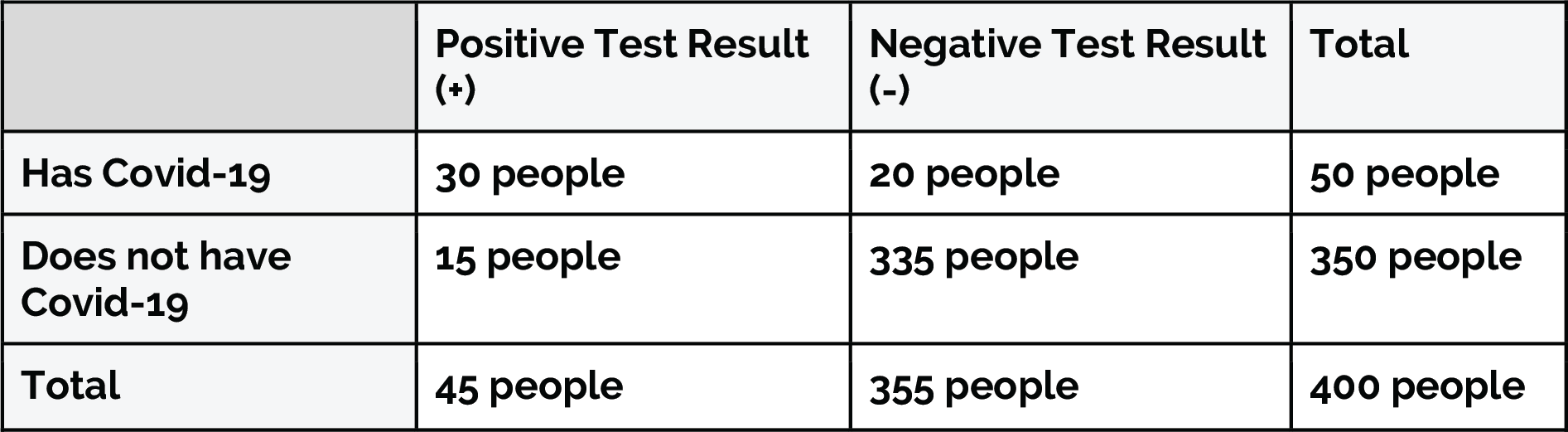# Sample Space

Sometimes it is helpful to list all of the possible ways that a chance process can turn out.

## Sample Space

In statistics, the sample space is the set of all possible outcomes of an event. For example, the sample space of rolling a fair six-sided die is shown below:

We can also look at the sample space for rolling two fair six-sided dice. The image below shows the 36 possible outcomes for rolling two fair six-sided dice.

Understanding the idea of sample space can help us calculate probabilities. We know that the probability of a certain outcome can be written as: P(outcome) = # of that outcome / total # of possibilities

The sample space can help us find the numerator and denominator of probabilities.

# Example Walk-Throughs with Worksheets

### Video 1: Sample Space and State Space

Follow along with the workseet to work through the problem:

# Practice Questions

Q1: P(A | B) is read as:
Q2: 400 people were tested for Covid-19 after attending a music festival. The results are shown in the table. What is the probability that you had Covid-19 given that you got a negative result (false negatives)? Use the table below.Q3: 400 people were tested for Covid-19 after attending a music festival. The results are shown in the table. What is the probability that you have Covid-19 given that you got a positive test result? Use the table below.Q4: 400 people were tested for Covid-19 after attending a music festival. The results are shown in the table. What is the probability that you got a negative test result given that you do not have Covid-19 (correct negatives)? Use the table below.Q5: 400 people were tested for Covid-19 after attending a music festival. The results are shown in the table. What is the probability that you get a positive test result given that you have Covid-19? Use the table below.